Download Presentation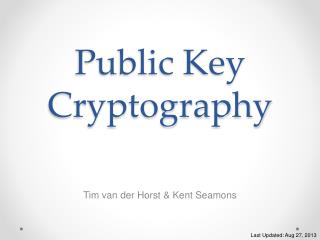Public Key Cryptography

# Public Key Cryptography - PowerPoint PPT Presentation

Public Key Cryptography. Tim van der Horst & Kent Seamons. Last Updated: Aug 27, 2013. Asymmetric Encryption. Why Public Key Crypto is Cool. Has a linear solution to the key distribution problem Symmetric crypto has an exponential solutionI am the owner, or an agent authorized to act on behalf of the owner, of the copyrighted work described.
Download Presentation## Public Key Cryptography

An Image/Link below is provided (as is) to download presentation

Download Policy: Content on the Website is provided to you AS IS for your information and personal use and may not be sold / licensed / shared on other websites without getting consent from its author.While downloading, if for some reason you are not able to download a presentation, the publisher may have deleted the file from their server.

- - - - - - - - - - - - - - - - - - - - - - - - - - E N D - - - - - - - - - - - - - - - - - - - - - - - - - -
Presentation Transcript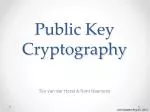### Public Key Cryptography

Tim van der Horst & Kent Seamons

Last Updated: Aug 27, 2013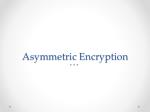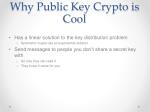Why Public Key Crypto is Cool
• Has a linear solution to the key distribution problem
• Symmetric crypto has an exponential solution
• Send messages to people you don’t share a secret key with
• So only they can read it
• They know it came for you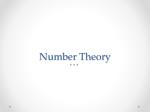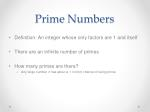Prime Numbers
• Definition: An integer whose only factors are 1 and itself
• There are an infinite number of primes
• How many primes are there?
• Any large number n has about a 1 in ln(n) chance of being primePrime Number Questions*
• If everyone needs a different prime number won’t we run out?
• Approximately 10151 primes 512 bits (or less)
• Atoms in the universe: 1077
• If every atom in the universe needed 1 billion primes every microsecond from the beginning of time until now you would need 10109 primes
• That means there’s still about 10151 left
• What if two people pick the same prime?
• Odds are significantly less than the odds of your computer spontaneously combusting at the exact moment you win the lotto

Source: Applied Cryptography (Schneier)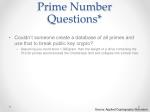Prime Number Questions*
• Couldn’t someone create a database of all primes and use that to break public key crypto?
• Assuming you could store 1 GB/gram, then the weight of a drive containing the 512-bit primes would exceed the Chandrasar limit and collapse into a black hole

Source: Applied Cryptography (Schneier)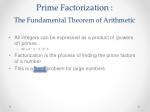Prime Factorization :The Fundamental Theorem of Arithmetic
• All integers can be expressed as a product of (powers of) primes
• 48 = 2 * 2 * 2 *2* 3
• Factorization is the process of finding the prime factors of a number
• This is a hard problem for large numbers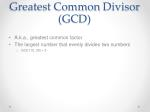Greatest Common Divisor (GCD)
• A.k.a., greatest common factor
• The largest number that evenly divides two numbers
• GCD (15, 25) = 5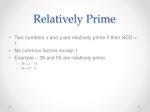Relatively Prime
• Two numbers x and y are relatively prime if their GCD = 1
• No common factors except 1
• Example – 38 and 55 are relatively prime
• 38 = 2 * 19
• 55 = 5 * 11Modular (%) Arithmetic
• Sometimes referred to as
• “clock arithmetic” or
• “arithmetic on a circle”
• Two numbers a and b are said to be congruent (equal) modulo N iffN/(a-b)
• Their difference is divisible by N with no remainder
• Their difference is a multiple of N
• a%n b%n
• Example – 30 and 40 are congruent mod 10
• Modulo operation
• Find the remainder (residue) 15 mod 10 = 5Notation
• Z - the set of integers {…-2,-1,0,1,2…}
• Zn - the set of integers modulo n; {0..n-1}
• Zn* - the multiplicative group of integers modulo n
• the set of integers modulo n that are relatively prime to n
• Zn* is closed under multiplication mod n
• Zn* does not contain 0 since the GCD(0,n)=n
• Z10* = ?
• Z12* = ?
• Z14* = ?• In Z, the additive inverse of 3 is -3, since 3 + -3 = 0, the additive identity.
• In Zn, the additive inverse of a is n-a, since a+(n-a) = n, which is congruent to 0 (mod n).
• What is the additive inverse of 4 mod 10?Multiplicative Inverse
• In Z, the multiplicative inverse of 3 is 1/3, since 3*1/3=1
• The multiplicative identity in both Z and Zn is 1
• The multiplicative inverse of 3 mod 10 is 7, since 3*7=21=1 (mod 10)
• This could be written 3-1, or (rarely) 1/3Distributive Property
• Distribution in + and *
• Modular arithmetic is distributive.

a+b (mod n) = (a mod n) + (b mod n) (mod n)Proof of Distributive Property
• Let a=cn+d. Then a%n=d, the remainder after taking out the multiples of n.
• Let b=en+f. Then b%n = f.a + b (mod n= cn+d + en+f (mod n) but cn = en = 0 (mod n) (since c and e are multiples of n), so:= d + f (mod n)
• = a%n + b%n (mod n).Proof of Distributive Property
• The modulus also distributes into multiplication. Consider a*b%n. Let a=cn+d and b=en+f, just as before. a * b (mod n)
• = (cn+d) * (en+f) (mod n) = cnen + cnf + den + df (mod n) = (cen)n + (cf)n + (de)n + df (mod n) But any multiple of n modulo n is 0, so = 0 + 0 + 0 + df (mod n) = a%n * b%n (mod n)Proof of Distributive Property
• An example helps: 7 * 26 (mod 5) = (1*5 + 2) * (5*5 + 1) (mod 5) = 1*5*5*5 + 1*5*1 + 2*5*5 + 2*1 (mod 5) = 0 + 0 + 0 + 2*1 (mod 5) = 7%5 * 26%5 (mod 5) = 2 (mod 5)Big Examples

What is the sum of these numbers modulo 20?

1325104987134069812734109243861723406983176

1346139046817340961834764359873409884750983

3632462309486723465794078340898340923876314

3641346983862309587235093857324095683753245

+ 2346982743069384673469268723406982374936877Big Examples

What is the product of these numbers modulo 25?

1234659823572938572

2139582753931306947

1398173619384713413

2496827464249812355

2436781359183781379

* 1351839761361377050Modular Exponentiation
• Problems of the form c = be modmgiven base b, exponent e, and modulus m
• If b, e, and m are non-negative and b < m, then a unique solution c exists and has the property 0 ≤ c < m
• For example, 12 = 52 mod 13
• Modular exponentiation problems are easy to solve, even for very large numbers
• However, solving the discrete logarithm (finding e given c, b, and m) is believed to be difficultBrute Force Method
• The most straightforward method to calculating a modular exponent is to calculate be directly, then to take this number modulo m. Consider trying to compute c, given b = 4, e = 13, and m = 497:
• One could use a calculator to compute 413; this comes out to 67,108,864. Taking this value modulo 497, the answer c is determined to be 445.
• Note that b is only one digit in length and that e is only two digits in length, but the value be is 10 digits in length.
• In strong cryptography, b is often at least 256 binary digits (77 decimal digits). Consider b = 5 * 1076 and e = 17, both of which are perfectly reasonable values. In this example, b is 77 digits in length and e is 2 digits in length, but the value be is 1304 decimal digits in length. Such calculations are possible on modern computers, but the sheer enormity of such numbers causes the speed of calculations to slow considerably. As b and e increase even further to provide better security, the value be becomes unwieldy.
• The time required to perform the exponentiation depends on the operating environment and the processor. If exponentiation is performed as a series of multiplications, then this requires O(e) time to complete.

Source: wikipedia – modular exponentiationDiffieHellman Project
• Write your own modular exponentiation routine
• Use a bignum library
• Divide and conquer algorithm O(log e)Diffie-Hellman Key Exchange
• Allows two users to establish a secret key over an insecure medium without any prior secrets
• Two system parameters p and g.
• Public values that may be used by all the users in a system
• Parameter p is a large prime number
• Parameter g (usually called a generator) is an integer less than p, such that for every number n with 0 < n < p , there is a power k of g such that n = gk mod p

g is primitive rootDiffie-Hellman Key Exchange
• Suppose Alice and Bob want to establish a shared secret key
• Alice and Bob agree on or use public values p,g
• p is a large prime number
• g is a generator
• Alice generates a random private value a and Bob generates a random private value b where a and b are integers
• Alice and Bob derive their public values using parameters p and g and their private values
• Alice's public value = ga mod p
• Bob’s public value is gb mod p
• Alice and Bob exchange their public values
• Alice computes gba = (gb)a mod pBob computes gab = (ga)b mod p
• Since gab= gba = k, Alice and Bob now have a shared secret key kA Crowded Room of Mathematicians

a=50

550 % 47 = 14

3150 % 47 = 18

b=49

549 % 47 = 31

1449 % 47 = 18

g=5

P=47

14

31Why is DH Secure?
• Discrete logarithm problem
• Inverse of modular exponentiation
• c = be modm
• e is called the “discrete logarithm”
• Solving the discrete logarithm (finding e given c, b, and m) is believed to be difficultAttacks Against DH
• Diffie-Hellman Key Exchange is secure against a passive attacker
• How can an active attacker disrupt the protocol?
• Man in the middle
• Modify Alice/Bob public values as they are exchanged
• Replace with Eve’s public values
• Replace with the value 1
• Replace with h, where h has a small orderPractical Considerations
• Chose a safe prime p where p=2q+1 where q is also prime
• How big should p be?
• 2048 bits (Source: Cryptography Engineering, Ferguson et al.)
• Use p, q, and g for performance reasons (smaller subgroup)
• Check public values for security properties
• Public values not equal to 1
• Public values that do not belong in too small a group
• Hash final result of DH to generate a shared key for Alice/Bob
• How to fortify the protocol against active attackers?
• Create a certified list of public values
• Use digitally signed public parameters